login  home  contents  what's new  discussion  bug reports help  links  subscribe  changes  refresh  edit

On Sunday, March 19, 2006 5:54 AM Francois Maltey wrote:

fricas
resFloat   : Expression Float := cos (4.0 * x)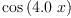(1)
Type: Expression(Float)
fricas
resInteger : Expression Integer := cos (4 * x)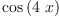(2)
Type: Expression(Integer)
fricas
resComplex : Expression Complex Integer := exp (3+4*%i)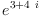(3)
Type: Expression(Complex(Integer))

How can I get Float from resFloat ? Integer from resInteger, etc. in a *.input file ?

I don't try to get the sub-argument, but the type of the subargument.

Imagine I know that a is an Expression... My function don't know if a is an Expression Float, or an Expression Integer...

I want to do as below in an *.input file:

  Myfunction (a : Expression ...) : String ==
is a an Expression Integer => "Integer"   -- or an other calculus.
is a an Expression Franction Integer => "Rationnal"
is a an Expression Complex ... => "Complex"   -- so I can use real and imag.
is a an Expression Float => "Float"
"A rare domain"


On Monday, March 20, 2006 7:53 PM William Sit wrote:

This is a bit tricky, since any function in Axiom must have a specific domain as source. The Axiom domain ANY is a coverall. Here is a try:

fricas
)set function compile on
Myfunction(a:Any):String ==
"A rare domain"
Function declaration Myfunction : Any -> String has been added to
workspace.
Type: Void

To use the function, coerce the input to Any, such as:

fricas
Myfunction(resInteger::Any)
fricas
Compiling function Myfunction with type Any -> String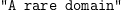(4)
Type: String

building on William Sit's ideas --Bill Page, Mon, 20 Mar 2006 20:36:07 -0600 reply
Based on what William suggested above, here is the way I might write the kind of function you want.

dom returns the domain of any expression as an "SExpression?" (SEX). An S-expression is the form used by Lisp and it can represent any expression as a special kind of list.

fricas
MyFunc2(a:ANY):OutputForm==
if dom(a).1 = "Expression"::Symbol::SEX then
dom(a).2 = ["Integer"::Symbol::SEX]::SEX =>
"Integer"
dom(a).2 = ["Float"::Symbol::SEX]::SEX =>
"Float"
dom(a).2 = ["Complex"::Symbol::SEX,["Integer"::Symbol::SEX]::SEX]::SEX =>
"Complex"
"A rare domain"
else
return hconcat(message("Can not handle: ")$OutputForm,(dom a)::OutputForm) Function declaration MyFunc2 : Any -> OutputForm has been added to workspace. Type: Void fricas MyFunc2(resFloat) fricas Compiling function MyFunc2 with type Any -> OutputForm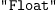(5) Type: OutputForm? fricas MyFunc2(resInteger)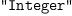(6) Type: OutputForm? fricas MyFunc2(resComplex)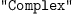(7) Type: OutputForm? fricas MyFunc2(1)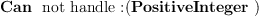(8) Type: OutputForm? fricas MyFunc2(x::EXPR FRAC INT)(9) Type: OutputForm? But, now a word of caution Is this really what you want to do? In generally, in Axiom we would like to use automatic polymorphism to select the appropriate function. In SPAD the "same" function can be defined many times using different signatures. So for example:  plus(x::Expression Float):Expression Float == ...  and:  plus(x::Expression Integer):Expression Integer == ...  can define different behaviours for the function plus depending on the type of the argument. Unfortunately it is not possible to do this in the Axiom interpreter. Here is another way using Union and case --Bill Page, Mon, 20 Mar 2006 21:12:25 -0600 reply Often you can do what you want to do by using the Union type. In some ways it is similar to Any but more specific. This allows you to use the case operation to test membership in the 'Union': fricas EI ==> Expression Integer Type: Void fricas EF ==> Expression Float Type: Void fricas ECI ==> Expression Complex Integer Type: Void fricas MyFunc3(a:Union(EI, EF, ECI)):String == a case EI => "Integer"; a case EF => "Float"; "Complex Integer" Function declaration MyFunc3 : Union(Expression(Integer),Expression( Float),Expression(Complex(Integer))) -> String has been added to workspace. Type: Void fricas MyFunc3(resFloat) fricas Compiling function MyFunc3 with type Union(Expression(Integer), Expression(Float),Expression(Complex(Integer))) -> String(10) Type: String fricas MyFunc3(resInteger)(11) Type: String fricas MyFunc3(resComplex)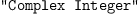(12) Type: String fricas MyFunc3(n::EXPR FRAC INT)(13) Type: String simpler version using ANY --Bill Page, Tue, 21 Mar 2006 00:48:57 -0600 reply We don't need those complicated looking S-expressions. It is easier just to generate them from a local variable. fricas MyFunc4(a:ANY):String== myEI:Expression Integer myEF:Expression Float myECI:Expression Complex Integer myEFI:Expression Fraction Integer dom(a) = dom(myEI) => "Integer" dom(a) = dom(myEF) => "Float" dom(a) = dom(myEFI) => "Rational" dom(a) = dom(myECI) => "Complex" "A rare domain" Function declaration MyFunc4 : Any -> String has been added to workspace. Type: Void fricas MyFunc4(resFloat) fricas Compiling function MyFunc4 with type Any -> String(14) Type: String fricas MyFunc4(resInteger)(15) Type: String fricas MyFunc4(resComplex)(16) Type: String fricas MyFunc4(1)(17) Type: String fricas MyFunc4(x::EXPR FRAC INT)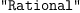(18) Type: String By studying http://wiki.axiom-developer.org/axiom--test--1/src/algebra/AnySpad we can see how to write this in Spad. Note that the function devaluate returns an S-expression that represents a domain. spad )abbrev package MYDOM MyDomain MyDomain: with MyFunc:Any->String == add MyFunc(a:Any):String== myEI==>Expression Integer myEF==>Expression Float myECI==>Expression Complex Integer myEFI==>Expression Fraction Integer dom(a) = devaluate(myEI)$Lisp => "Integer"
dom(a) = devaluate(myEF)$Lisp => "Float" dom(a) = devaluate(myEFI)$Lisp => "Rational"
dom(a) = devaluate(myECI)$Lisp => "Complex" "A rare domain" spad  Compiling FriCAS source code from file /var/lib/zope2.10/instance/axiom-wiki/var/LatexWiki/7109084728131837944-25px010.spad using old system compiler. MYDOM abbreviates package MyDomain ------------------------------------------------------------------------ initializing NRLIB MYDOM for MyDomain compiling into NRLIB MYDOM compiling exported MyFunc : Any -> String processing macro definition myEI ==> Expression Integer processing macro definition myEF ==> Expression Float processing macro definition myECI ==> Expression Complex Integer processing macro definition myEFI ==> Expression Fraction Integer Time: 0.06 SEC. (time taken in buildFunctor: 0) ;;; *** |MyDomain| REDEFINED ;;; *** |MyDomain| REDEFINED Time: 0.01 SEC. Cumulative Statistics for Constructor MyDomain Time: 0.07 seconds finalizing NRLIB MYDOM Processing MyDomain for Browser database: --->-->MyDomain(constructor): Not documented!!!! --->-->MyDomain((MyFunc ((String) (Any)))): Not documented!!!! --->-->MyDomain(): Missing Description ; compiling file "/var/aw/var/LatexWiki/MYDOM.NRLIB/MYDOM.lsp" (written 04 APR 2022 05:25:52 PM): ; /var/aw/var/LatexWiki/MYDOM.NRLIB/MYDOM.fasl written ; compilation finished in 0:00:00.012 ------------------------------------------------------------------------ MyDomain is now explicitly exposed in frame initial MyDomain will be automatically loaded when needed from /var/aw/var/LatexWiki/MYDOM.NRLIB/MYDOM fricas MyFunc(resInteger)$MyDomain(19)
Type: String
fricas
MyFunc(x::EXPR FRAC INT)\$MyDomain(20)
Type: String

 Subject: (replying)   Be Bold !! ( 14 subscribers )